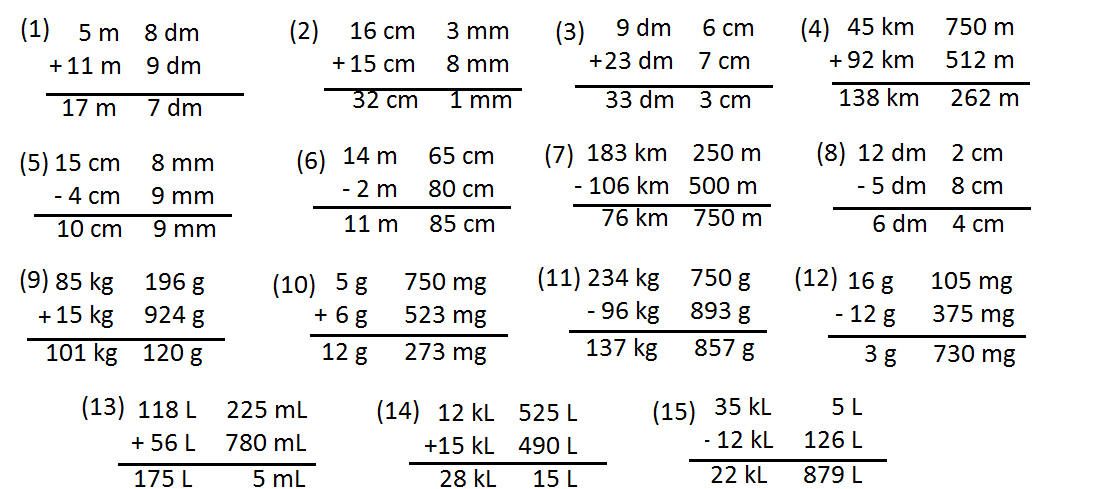# S. K. Gupta Anubhuti Gangal Class 5 Math Ninth Chapter Measurement Exercise 37

## EXERCISE 37(16) Find in meters the height of a pile of 25 books if each book is 3 cm 5 mm thick.

Solution: Thickness of one book = 3 cm 5 mm = 3.5 cm

∴ Thickness of 25 books = (3.5 × 25) = 87.5 cm = 87 cm 50 mm

(17) Ritu drew a line segment of length 15 cm 4 mm. Then she erased a portion of it. The remaining line segment measured 7 cm 6 mm. What is the length in mm of the erased line segment?

Solution: Length of line which ritu drew,

= 15 cm 4 mm = (15 × 100) + 4 mm = 1504 mm

Ritu erased the portion = 7 cm 6 mm = (7 × 100) + 6 = 706 mm

∴ The length of the erased line segment = (1504 – 706) mm = 798 mm.

(18) Vivek jogs 8 times around a square field of 140 m. How many kilometers and meters does he jog? He had planned to jog 1.5 km. How much in meters is still left jog.

Solution: Vivek jogs 1 time = 140 m.

∴ Vivek jogs 8 times = (140 × 8) m = 1120 m. = 1.120 km

If he planned to jog 1.5 km, so he still left jog = (1.5 – 1.120) km = 0.38 km = (0.38 × 1000) m = 380 m.

(19) Manish’s weight is 83 kg 450 g and Ashish is 6 kg 900 g heavier than him. Find Ashish’s weight in kg.

Solution: Manish’s weight = 83 kg 450 g

Ashish is heavier than Manish = 6 kg 900 g

∴ Ashis’s weight = (83 kg 450 g + 6 kg 900 g) = 90 kg 350 g.

(20) Find the net weight in grams of the contents of a breakfast cereal package if the gross weight is 1 kg 200 g and the packaging weighs 15 g.

Solution: The gross weight of the packet = 1 kg 200 g = (1 × 1000) + 200 g = 1200 g

The packaging weigh = 15 g

∴ The net weight of the packet = (1200 – 15) g = 1185 g = 1 kg 185 g.

(21) 15 bags of wheat were delivered at a grocery shop, the total being 144 kg 780 g. If all the bags contain equal amount of wheat, find the weight of each bag?

Solution: The weight of 15 bag = 144 kg 780 g = (144 × 1000) + 780 g = 144780 g

∴ The weight of 1 bag of wheat = (144780 ÷ 15) = 9652 g = 9 kg 652 g.

(22) For a birthday party a 10 L oil can was purchased. After the party 6 L 450 mL of oil was still left in the can. How much oil in liters was in the party?

Solution: The amount of purchased oil = 10 L = 10000 mL

The left oil of after birthday party = 6 L 450 mL = (6 × 1000) + 450 ml = 6450 mL

∴ Uses oil in the party = (10,000 – 6450) mL = 3550 mL = 3 L 550 mL.

(23) How many 150 mL glasses can I fill with 5 bottles of soft drinks each holding 1.2 liters?

Solution: Each bottle can hold = 1.2 L = (1 × 1000) + 200 mL = 1200 mL

Amount of 5 bottles = (1200 × 5) mL = 6000 mL

∴ 150 mL glasses can fill = (6000 ÷ 150) = 40 glasses.

(24) A bottle holds 1 L 140 mL of shampoo, Radhika uses 95 mL of shampoo each week.

(a) How many weeks will the bottle of shampoo last?

Solution: The amount of shampoo of the bottle = 1 L 140 mL = (1 × 1000) +140 mL = 1140 mL

Radhika use shampoo in each week = 95 mL

∴ The required weeks = (1140 ÷ 95) = 12 weeks.

(b) If she accidently spills 475 mL of shampoo, how long will the bottle last?

Solution: If she accidently spill 475 mL shampoo then the bottle had = (1140 – 475) mL = 665 mL shampoo

Then the required week = (665 ÷ 95) = 7 weeks.

Updated: May 31, 2022 — 12:45 pm